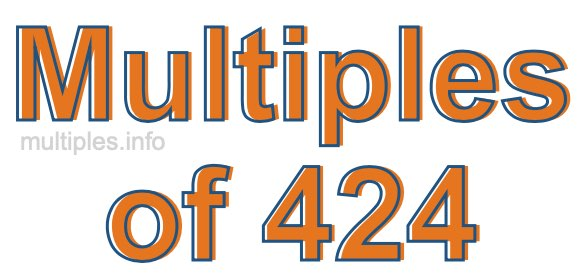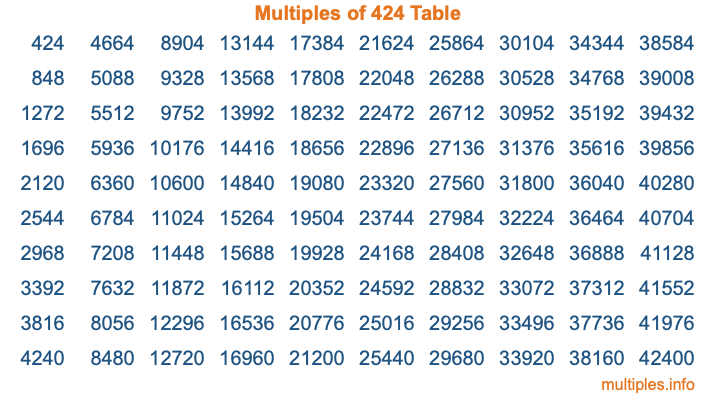Multiples of 424Welcome to the Multiples of 424 page. Here we will first teach you everything you will ever need to know about the multiples of 424, and then give you a study guide summary of everything we taught you to make sure you remember it all. Use this page to look up facts and learn information about the multiples of 424. This page will make you a multiples of four hundred twenty-four expert!

Definition of Multiples of 424
Multiples of 424 are all the numbers that when divided by 424 equal an integer. Each of the multiples of 424 are called a multiple. A multiple of 424 is created by multiplying 424 by an integer.

Therefore, to create a list of multiples of 424, you start with 1 multiplied by 424, then 2 multiplied by 424, then 3 multiplied by 424, and so on for as long as you want. Thus, the list of the first five multiples of 424 is 424, 848, 1272, 1696, and 2120. To see a larger list of multiples of 424, see the printable image of Multiples of 424 further down on this page. We also have a category where you can choose any nth multiple of 424.

Multiples of 424 Checker
The Multiples of 424 Checker below checks to see if any number of your choice is a multiple of 424. In other words, it checks to see if there is any number (integer) that when multiplied by 424 will equal your number. To do that, we divide your number by 424. If the the quotient is an integer, then your number is a multiple of 424.

Is  a multiple of 424?

Least Common Multiple of 424 and ...
A Least Common Multiple (LCM) is the lowest multiple that two or more numbers have in common. This is also called the smallest common multiple or lowest common multiple and is useful to know when you are adding our subtracting fractions. Enter one or more numbers below (424 is already entered) to find the LCM.

Check out our LCM Calculator if you need more details about the Least Common Multiple or if you need the LCM for different numbers for adding and subtraction fractions.

nth Multiple of 424
As we stated above, 424 is the first multiple of 424, 848 is the second multiple of 424, 1272 is the third multiple of 424, and so on. Enter a number below to find the nth multiple of 424.

th multiple of 424

Multiples of 424 vs Factors of 424
424 is a multiple of 424 and a factor of 424, but that is where the similarities end. All postive multiples of 424 are 424 or greater than 424. All positive factors of 424 are 424 or less than 424.

Below is the beginning list of multiples of 424 and the factors of 424 so you can compare:

Multiples of 424: 424, 848, 1272, 1696, 2120, etc.

Factors of 424: 1, 2, 4, 8, 53, 106, 212, 424

As you can see, the multiples of 424 are all the numbers that you can divide by 424 to get a whole number. The factors of 424, on the other hand, are all the whole numbers that you can multiply by another whole number to get 424.

It's also interesting to note that if a number (x) is a factor of 424, then 424 will also be a multiple of that number (x).

Multiples of 424 vs Divisors of 424
The divisors of 424 are all the integers that 424 can be divided by evenly. Below is a list of the divisors of 424.

Divisors of 424: 1, 2, 4, 8, 53, 106, 212, 424

The interesting thing to note here is that if you take any multiple of 424 and divide it by a divisor of 424, you will see that the quotient is an integer.

Multiples of 424 Table
Below is an image of the first 100 multiples of 424 in a table. The table is in chronological order, column by column. The first column has the first ten multiples of 424, the second column has the next ten multiples of 424, and so on.The Multiples of 424 Table is also referred to as the 424 Times Table or Times Table of 424. You are welcome to print out our table for your studies.

Negative Multiples of 424
Although not often discussed or needed in math, it is worth mentioning that you can make a list of negative multiples of 424 by multiplying 424 by -1, then by -2, then by -3, and so on, to get the following list of negative multiples of 424:

-424, -848, -1272, -1696, -2120, etc.

Multiples of 424 Summary
Below is a summary of important Multiples of 424 facts that we have discussed on this page. To retain the knowledge on this page, we recommend that you read through the summary and explain to yourself or a study partner why they hold true.

There are an infinite number of multiples of 424.

A multiple of 424 divided by 424 will equal a whole number.

424 divided by a factor of 424 equals a divisor of 424.

The nth multiple of 424 is n times 424.

The largest factor of 424 is equal to the first positive multiple of 424.

424 is a multiple of every factor of 424.

424 is a multiple of 424.

A multiple of 424 divided by a divisor of 424 equals an integer.

424 divided by a divisor of 424 equals a factor of 424.

Any integer times 424 will equal a multiple of 424.

Multiples of a Number
Here you can get the multiples of another number, all with the same attention to detail as we did for multiples of 424 on this page.

Multiples of
Multiples of 425
Did you find our page about multiples of four hundred twenty-four educational? Do you want more knowledge? Check out the multiples of the next number on our list!